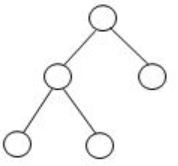## GATE 1987

 Question 1

In a microprocessor-based system, if a bus (DMA) request and an interrupt request arrive simultaneously, the microprocessor attends first to the bus request.

 A True. B False.
Computer-Organization       Modes-of-Transfer       Video-Explanation
Question 1 Explanation:
The HOLD input has a higher priority than the INTR or NMI interrupt inputs.
 Question 2

Data transfer between a microprocessor and an I/O device is usually faster in memory-mapped-I/O scheme than in I/O-mapped - I/O scheme.

 A True B False
Computer-Organization       I/O-Handling       Video-Explanation
Question 2 Explanation:
Memory mapped I/0 runs faster than I/0 mapped I/O.
 Question 3

It is possible to construct a binary tree uniquely whose pre-order and post-order traversals are given?

 A True B False
Data-Structures       Binary-Trees       Video-Explanation
Question 3 Explanation:
To construct binary tree uniquely we need either inorder and postorder or inorder and preorder.
 Question 4

The union of two equivalence relations is also an equivalence relation.

 A True B False
Engineering-Mathematics       Sets-And-Relation       Video-Explanation
Question 4 Explanation:
Equivalence relation should satisfy Reflexive, Symmetric and Transitive property.
Reflexive ∪ Reflexive = Reflexive
Symmetric ∪ Symmetric = Symmetric
Transitive ∪ Transitive ≠ Transitive
Since Transitivity does not satisfy union, so union of two equivalence relation is not an equivalence relation.
 Question 5

There is a linear-time algorithm for testing the planarity of finite graphs.

 A True B False
Data-Structures       Graphs       Video-Explanation
Question 5 Explanation:
Yes, Linear time algorithm is there for testing the planarity of finite graphs.
 Question 6

Every infinite cyclic group is isomorphic to the infinite cyclic group of integers under addition.

 A True B False
Engineering-Mathematics       Sets-And-Relation       Video-Explanation
Question 6 Explanation:
True, every infinite cyclic group is isomorphic to the infinite cyclic group of integers under addition.
 Question 7

If the number of leaves in a tree is not a power of 2, then the tree is not a binary tree.

 A True B False
Data-Structures       Binary-Trees       Video-Explanation
Question 7 Explanation:In the above binary tree, no. of leaves is 3, which is not the power of 2. Hence the given statement is false.
 Question 8

Regularity is preserved under the operation of string reversal.

 A True B False
Theory-of-Computation       Regular-Language       Video-Explanation
Question 8 Explanation:
Regular language is closed under reversal.
There are 8 questions to complete.

Register Now